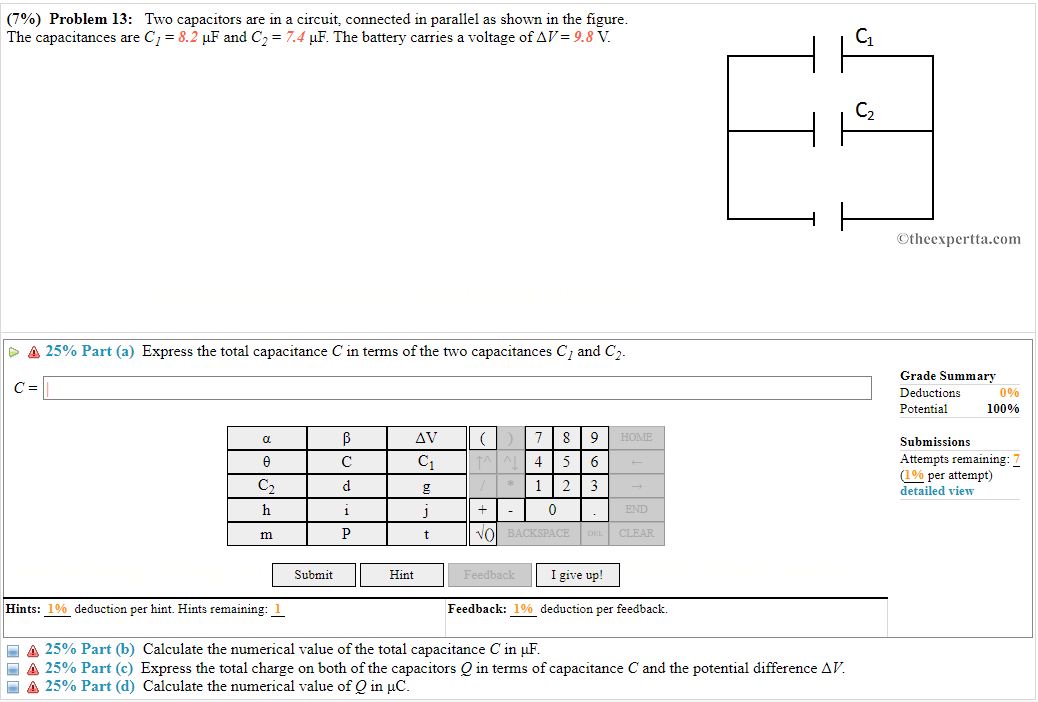Home / Expert Answers / Physics / 7-problem-13-two-capacitors-are-in-a-circuit-connected-in-parallel-as-shown-in-the-figure-t-pa422

# (Solved): (7\%) Problem 13: Two capacitors are in a circuit, connected in parallel as shown in the figure. T ...(7\%) Problem 13: Two capacitors are in a circuit, connected in parallel as shown in the figure. The capacitances are $$C_{1}=8.2 \mu \mathrm{F}$$ and $$C_{2}=7.4 \mu \mathrm{F}$$. The battery carries a voltage of $$\Delta V=9.8 \mathrm{~V}$$. $$\triangle 25 \%$$ Part (a) Express the total capacitance $$C$$ in terms of the two capacitances $$C_{1}$$ and $$C_{2}$$. $$C$$ Hints: deduction per hint. Hints remaining: Feedback: deduction per feedback. A 25\% Part (b) Calculate the numerical value of the total capacitance $$C$$ in $$\mu \mathrm{F}$$. $$\triangle 25 \%$$ Part (c) Express the total charge on both of the capacitors $$Q$$ in terms of capacitance $$C$$ and the potential difference $$\Delta V$$. $$\triangleq 25 \%$$ Part (d) Calculate the numerical value of $$Q$$ in $$\mu \mathrm{C}$$.

We have an Answer from Expert